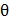# In diffraction from a single slit, the angular width of the central maxima does not depend on a)of light used b) Width of slit c) Distance of slits from screen d) Ratio ofand slit width

## Question ID - 150088 :- In diffraction from a single slit, the angular width of the central maxima does not depend on a)of light used b) Width of slit c) Distance of slits from screen d) Ratio ofand slit width

3537

(c)

For a slit of widthlight of wavelengthwhen light falls on the slit, the diffraction patterns so obtained asThe first diffraction minimum occurs at the angles given byFrom the equation, it is clear that width of the central diffraction maximum is inversely proportional to the width of the slit.  On increasing the width size, the angleat which the intensity first becomes zero decreases, resulting in a narrower central band and if the slit width is made smaller, the angleincreases, giving a wider central band.

Next Question :
 A wave can transmit ………. from one place to another a) Energy b) Amplitude c) Wavelength d) Matter